#### Date

25 Mar 2020

This week's assignment is aimed to modify our board that we have designed before, by adding a sensor and testing it.

I used the Arduino board temporarily instead of a new PCB, because of the curfew situation.

There are many types of input devices, such as ultrasonic sensor, motion Sensor, pressure Sensor and IR Sensor.

I decided to test two of the input devices :

• LDR

• Ultrasonic

#### LDR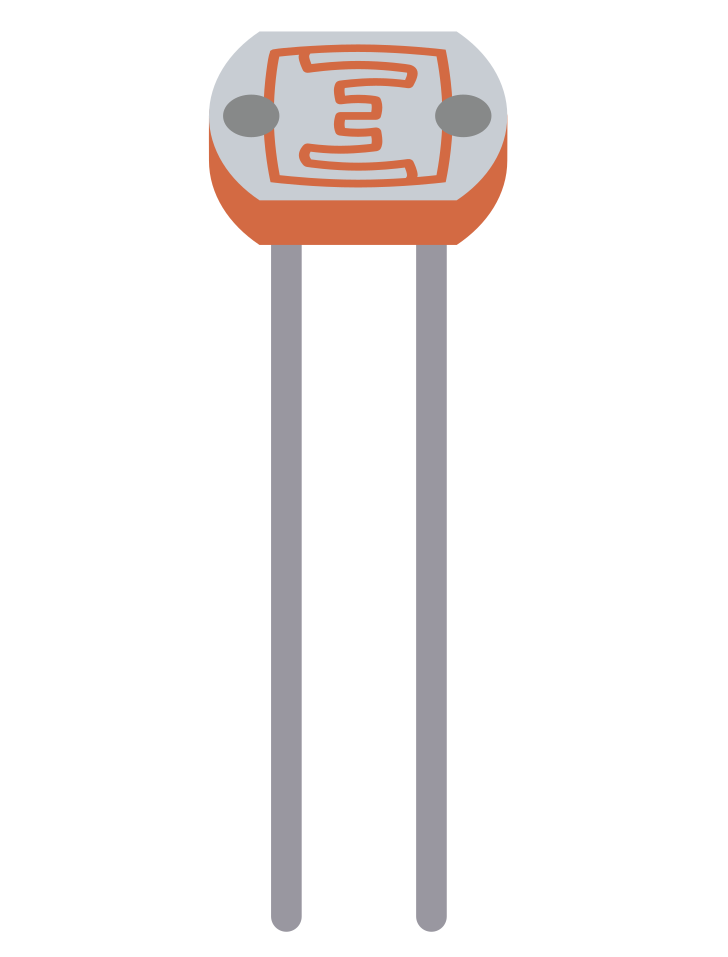#### What is LDR ?

A Light dependent Resistor known as a LDR, is a variable resistance that changes depending on the intensity of light that falls on its top surface. It has other names : photoconductor, photoresistor and photocell.

This resistance has an Inverse relationship with the light, when the light amount increases, the value of the resistance will decrease, and vice versa.

#### The main parts of LDR :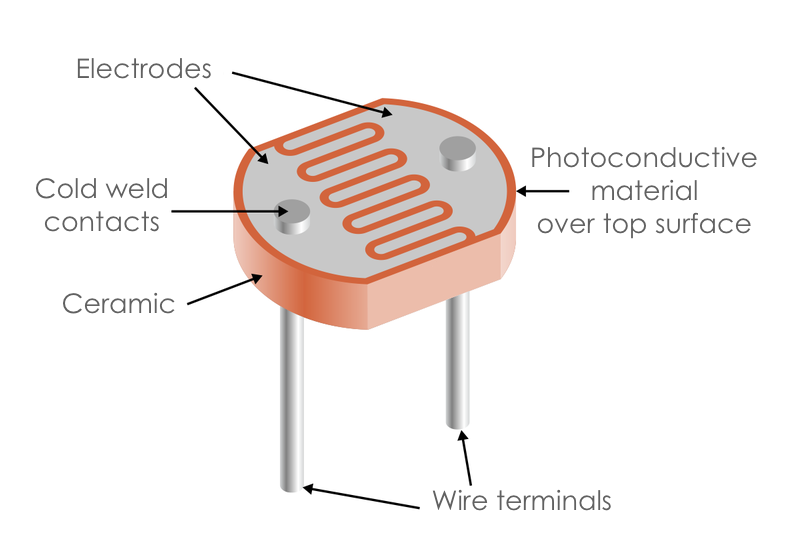There are many uses of LDR in everyday Life, for example:

• Alarm clocks

• Street lights

• Light intensity meters

• Burglar alarm circuits

To start, I Identified the components that I need to turn LED on and off through LDR.

The components list :

• 1 x Arduino board

• 7 x Jumper Wires

• 1 x LDR sensor

• 1 x LED

• 1 x 10k Ω Resistor

• 1 x 220 Ω Resistor

The 10k resistor used along with the LDR to create a voltage divider circuit. The LDR varying resistance is converted to a varying voltage which will be used by the analog pin.

Before connecting the components, I created a virtual circuit by using the Tinkercad simulator to check the connection and the code and avoid mistakes in the real circuit.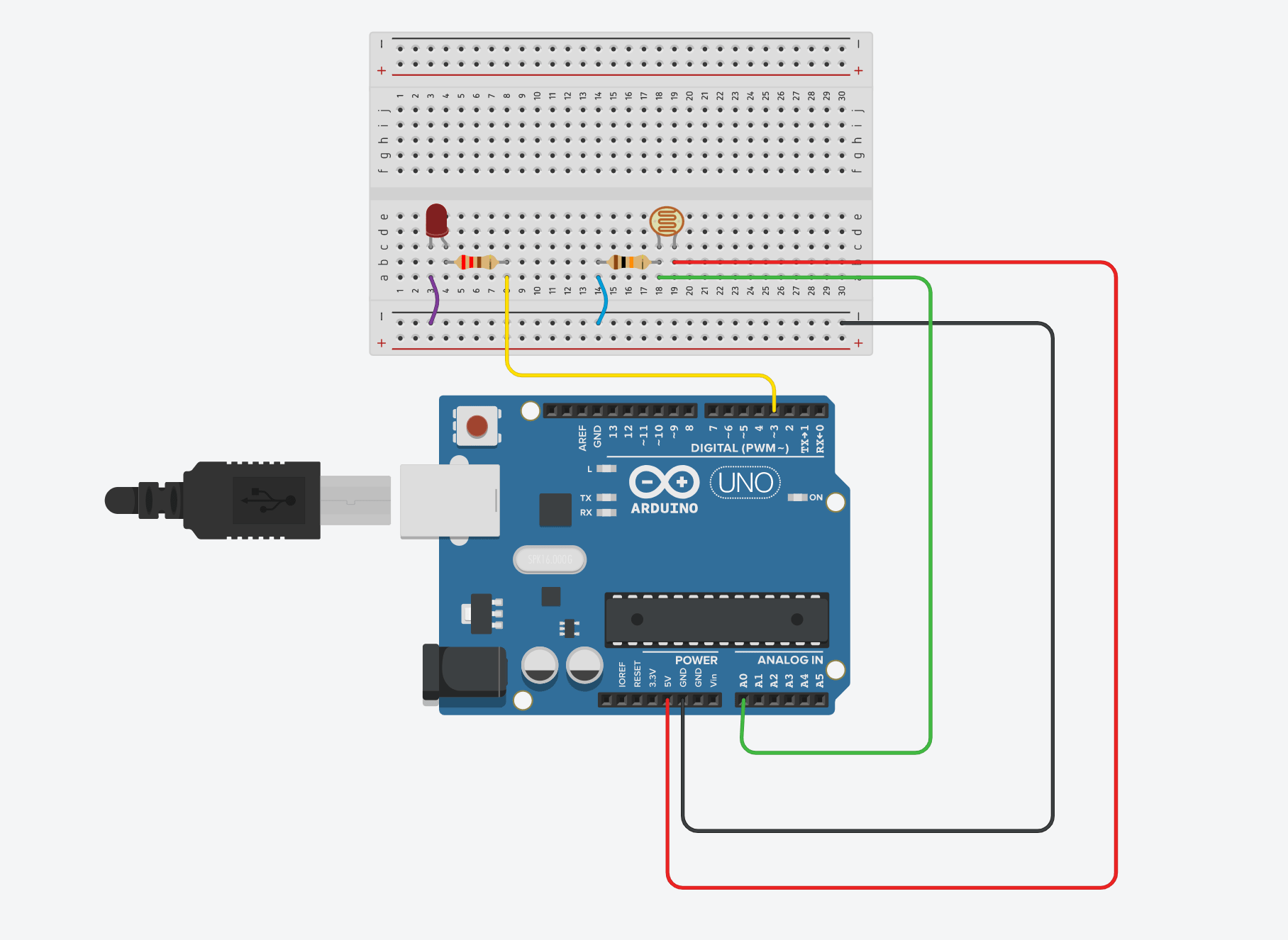After the simulation process, I started wireing up the co mponents by placing the LDR on the breadboard first. Then, I connected one of the LDR pins with the 10k Ω resistor to the analog input (A0) for reading the approximate value of the light levels.

The other leg off the LDR connected to the +5v. Next, I attached the 220 Ω resistor to the LED anode leg and the other resistor leg to the digital pin 13 of the Arduino. Lastly, the LED cathode leg connected to GND.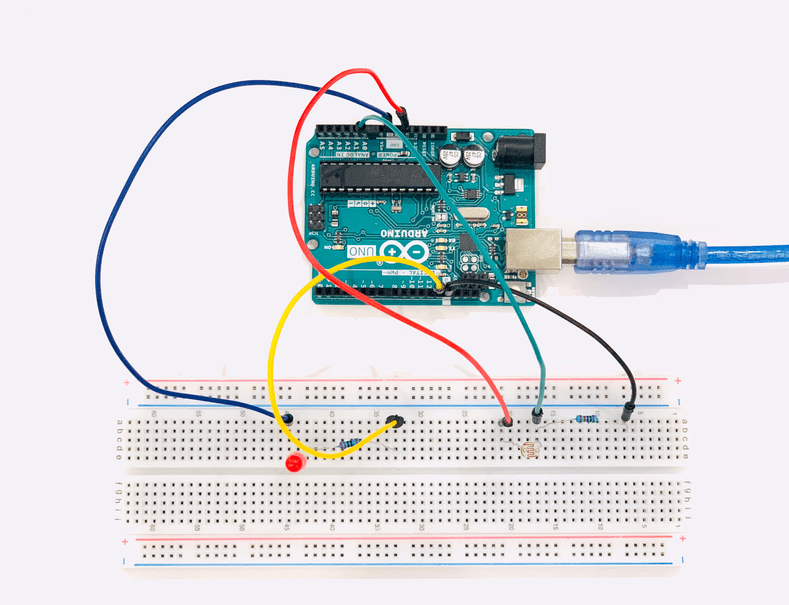Next, I opened the Arduino IDE and uploaded the code. In this test, the LDR will work as a switch. If I cover the photocell to be in darkness, the LED will turn on and if I keep the light falling on its surface, the LED will turn off.

Now that everything is ready, It is time for my favorite part, coding !

#### PROGRAMMING

• Define the LED pin and the LDR pin.

• `const int ledPin = 13;`

`const int ldrPin = A0;`

In the `void setup()` section:

• write the serial begin function which is used to set the data rate in bits per second (baud) for serial data transmission.

• `Serial.begin(9600);`

• Initialize the pin mode by making the LED the output and the LDR the input.

• `pinMode(ledPin, OUTPUT);`

`pinMode(ldrPin, INPUT); `

In the `void loop()` section:

• Use the analog read function to read the value of the LDR.

• `int ldrStatus = analogRead(ldrPin); `

• Use If else statement.

• If the status is less than or equal 300, the LED will turn ON.

` if (ldrStatus <=300) {`

`digitalWrite(ledPin, HIGH);`

• Display the status.

• `Serial.println("LDR is DARK, LED is ON");`

Else, the LED will turn off and display the status.

` } else { `

`digitalWrite(ledPin, LOW);`

`Serial.println("---------------")`

The code :

### It works !

#### Ultrasonic Sensor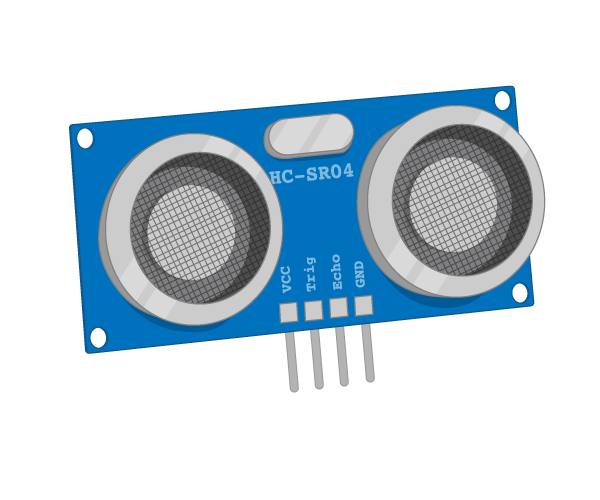#### What is Ultrasonic ?

An Ultrasonic sensor is a tool that measures the distance by sending an ultrasonic wave at a specific frequency and receiving the reflected wave from the object.

Ultrasonic sensor has four pins :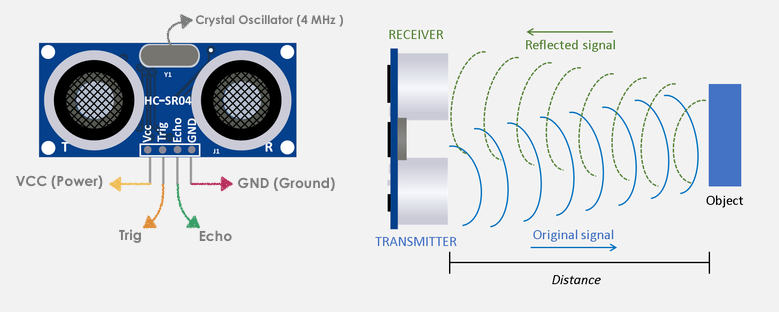• VCC pin is connected to +5V.

• Trig is an Input pin that sended a signal to trigger the Ultrasonic sound pulses.

• Echo is an Output pin that sended a signal back after detecting the object.

• GND pin connected to the ground.

The distance can be calculate using this formula :

Distance = Speed * Time/2

There are many applications of the Ultrasonic sensor in daily Life, for example:

• Vehicle detection for car wash.

• Anti-Collision Detection.

• Human Presence Detection.

• Pallet Detection with Forklifts.

I determined the components that I need to turn LED on or off by detecting the distance through the Ultrasonic sensor.

The components list :

• 1 x Arduino board

• 8 x Jumper Wires

• 1 x Ultrasonic sensor

• 1 x LED

• 1 x 1k Ω Resistor

To create a successful Arduino experiment, I started making the circuit using the Tinkercad simulator by dragging the components and connecting them to the breadboard and the Arduino board.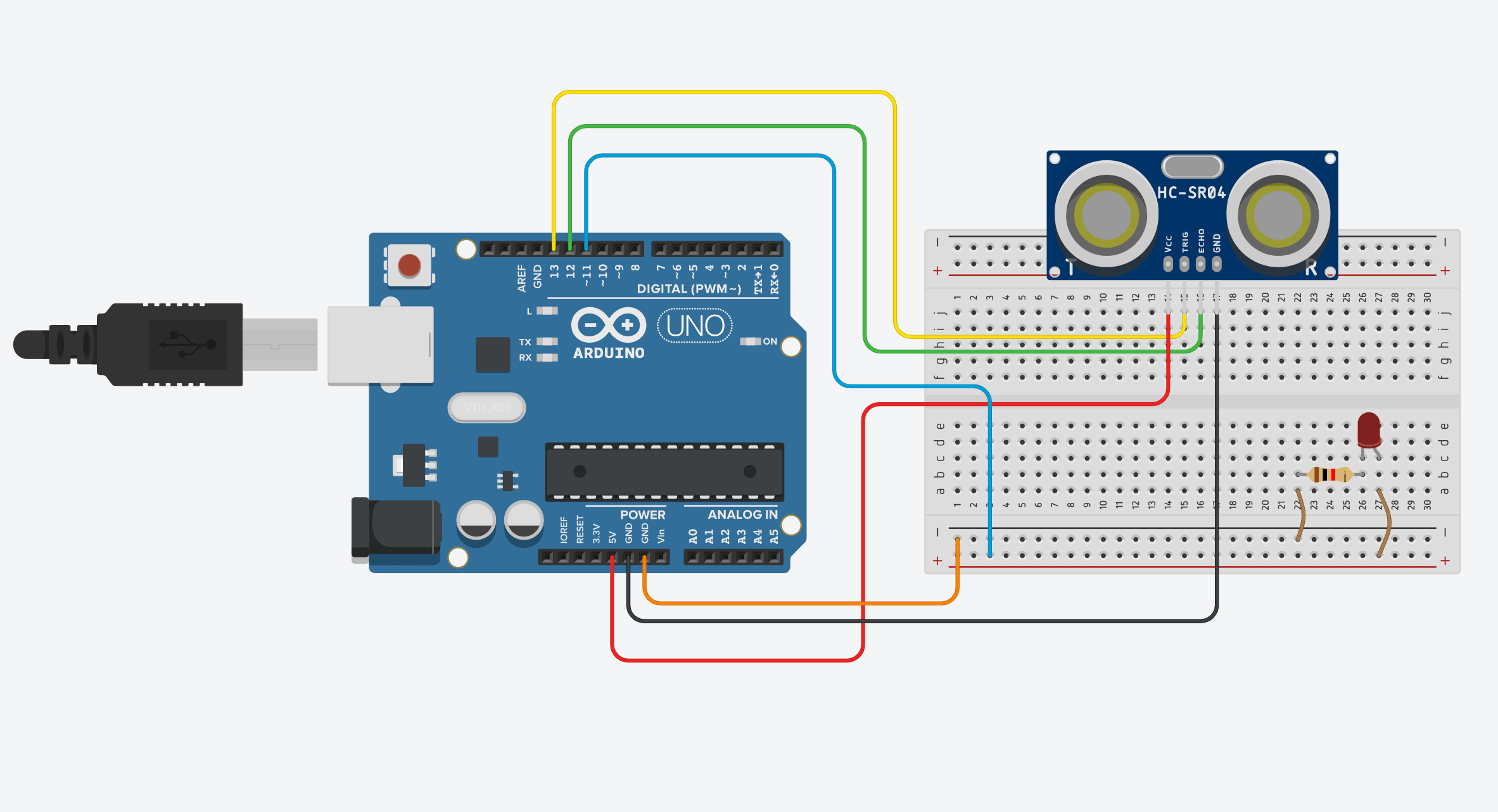After the simulation, I connected the physical components together. I started by placing the Ultrasonic to the breadboard. Next, connected the Ultrasonic HC-SR04 Pins distance sensor as the following :

Then, I attached the 1k Ω resistor to the LED cathode leg and the other resistor leg to the negative rail of the Arduino. Then, the LED anode leg connected to the positive rail.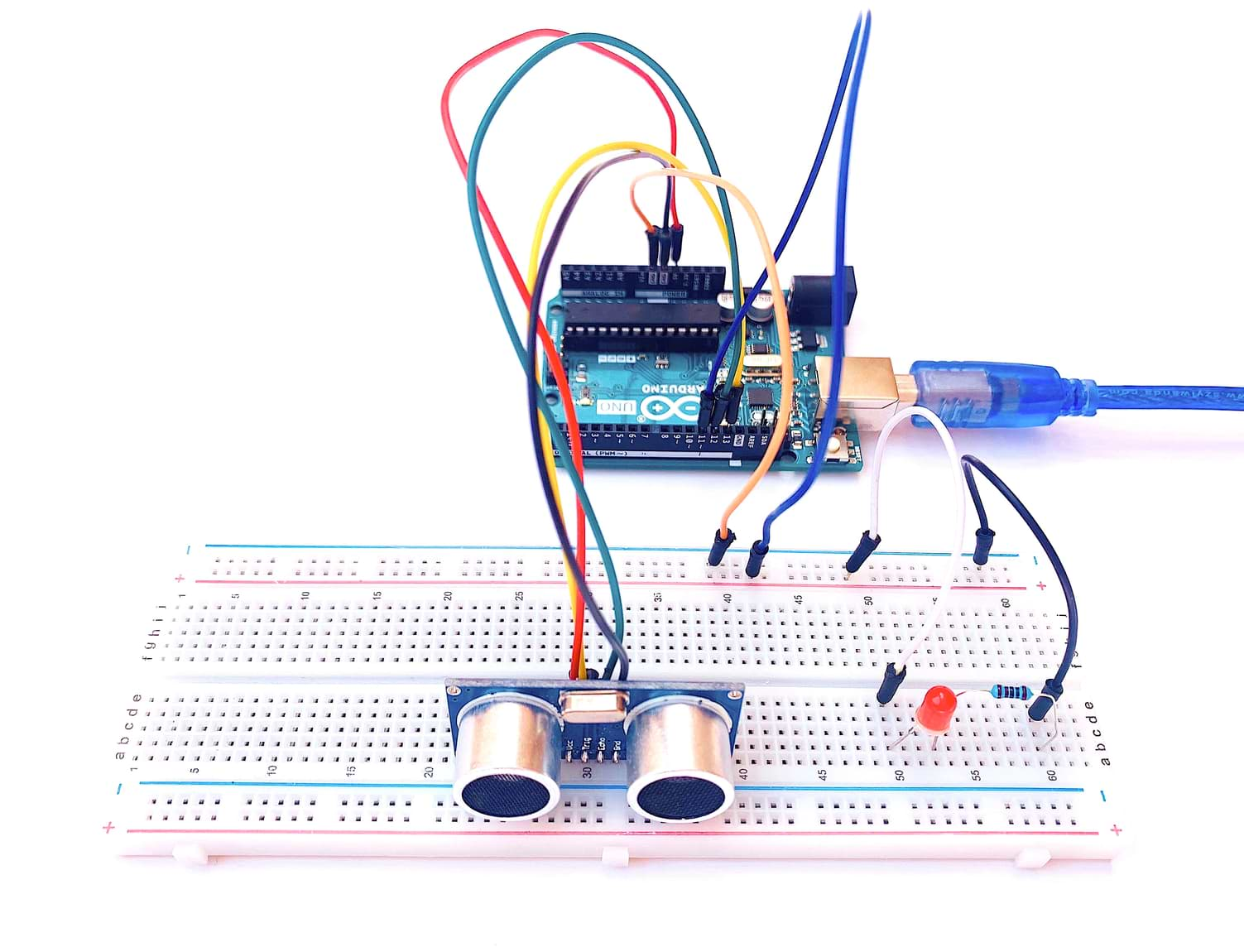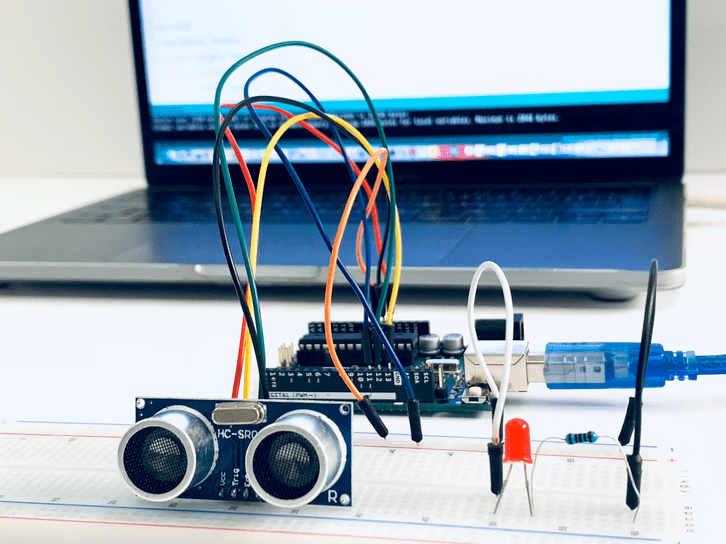#### PROGRAMMING

To write the code:

• Define pin numbers for the trig, echo and LED.

• `int trigPin = 13;`

`int echoPin = 12 ;`

`int led = 11 ;`

In the `void setup()` section:

• Write the serial begin function to show the result in the serial monitor.

• `Serial.begin (9600);`

• Define the pin mode by making the Echo as input, the LED and the Trig as output.

• `pinMode(trigPin, OUTPUT);`

`pinMode(echoPin, INPUT);`

`pinMode(led, OUTPUT);`

In the `void loop()` section:

• Defined variables by using `long` function which is extended size variables for number storage.

• `long duration, distance;`

• Set the Trig pin on a low state for 2 microseconds to make it clear.

• `digitalWrite(trigPin, LOW);`

`delayMicroseconds(2);`

• Generate the ultrasound wave I set the Trig pin on high state for 10 microseconds.

• `digitalWrite(trigPin, HIGH);`

`delayMicroseconds(10);`

`digitalWrite(trigPin, LOW);`

• Read travel time by using `pulseIn` function and put that value into the variable “duration”

• `duration = pulseIn(echoPin, HIGH);`

• Calculate the distance by by the following equation:

• `distance = (duration/2) / 29.1;`

The duration is divided by two because the sound has to travel the distance twice then divide it by 29.1 which is the speed of sound.

• Use If else statement :

• If the distance is less than 10 cm, the led will turn on.

`if (distance < 10)`

`if{ digitalWrite(led,HIGH);`

Else, the led will turn off.

`else {`

`digitalWrite(led,LOW);`

• Print the value of the distance on Serial Monitor.

• `Serial.print(distance);`

`Serial.println(" cm");`

• Use delay() which is a blocking function that prevents a program from doing anything else until that particular task has completed.

• `delay(500);`

The code:

### It works !

After all the exercises that I tried with the arduino board, finally the lockdown ended. I made my PCB with Atmega atmega328p microcontroller

#### What is ATMEGA328P microcontroller?

Atmega 328p from Atmel is a high performance, low power controller from Microchip. It is an 8-bit microcontroller based on AVR RISC architecture. It is the most popular of all AVR controllers as it is used in ARDUINO boards.

#### Atmega 328p features :

• High Performance, Low Power AVR® 8-Bit Microcontroller.

• Digtal 14 Pin I\O.

• Analog 6 Pin input.

• 131 Powerful Instructions – Most Single Clock Cycle Execution.

• 32 x 8 General Purpose Working Registers.

• Fully Static Operation.

• Up to 20 MIPS Throughput at 20 MHz.

• On-chip 2-cycle Multiplier.

#### Atmega 328p pins :

Pinout diagram is shown in below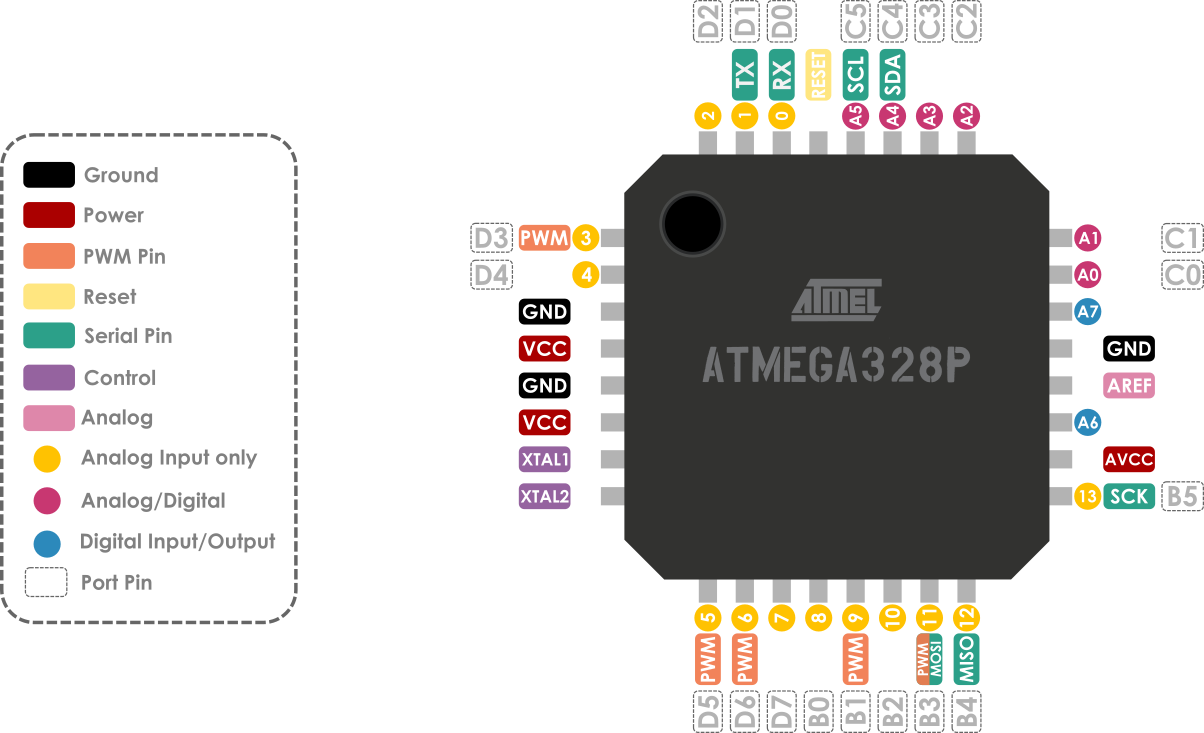#### RFID

RFID means radio-frequency identification, a technology used for electronic and wireless identification of objects, humans and animals. It uses electromagnetic fields to transfer data over short distances.

#### Specifications:

• Input voltage: 3.3V

• Frequency: 13.56MHz

• Communication : SPI, I2C protocol, UART

• Maximum Data Rate: 10Mbps

#### RFID pinout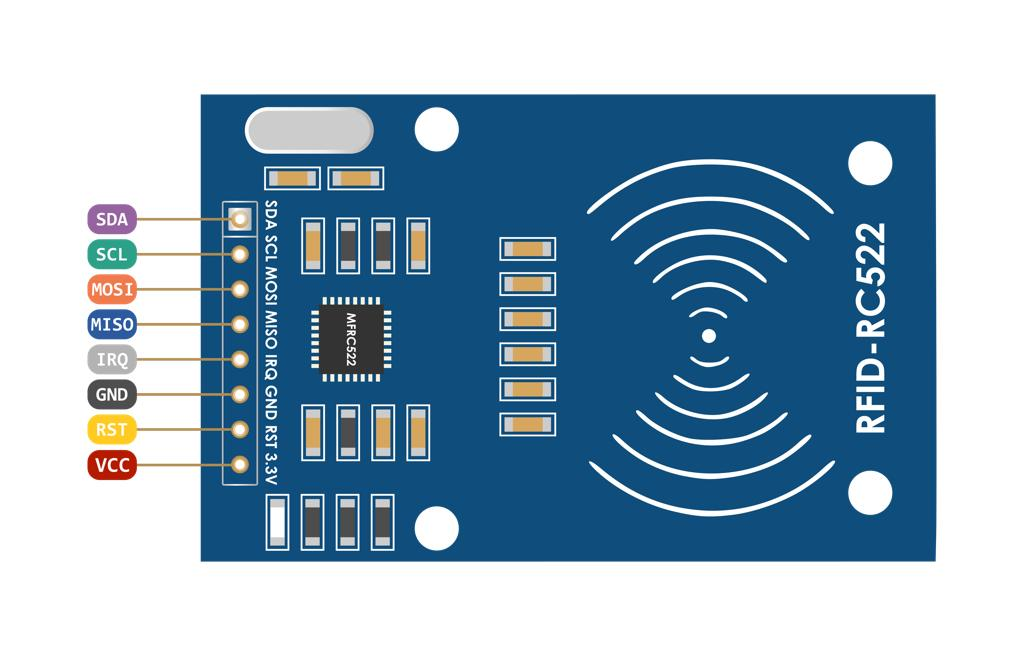• VCC supplies power for the module.

• RST is an input for Reset and power-down.

• GND is the Ground Pin.

• IRQ is an interrupt pin that can alert the microcontroller when RFID tag comes into its vicinity.

• MISO / SCL / Tx pin acts as Master-In-Slave-Out when SPI interface is enabled.

• MOSI (Master Out Slave In) is SPI input to the RC522 module.

• SCK (Serial Clock) accepts clock pulses provided by the Arduino.

• SS / SDA / Rx pin acts as Signal input when SPI interface is enabled.

Each RFID card has a unique ID to present a specific command. I used a simple code to prepare RFID Cards and test it.

#### Components

• Arduino UNO

• RFID Tags

• LEDs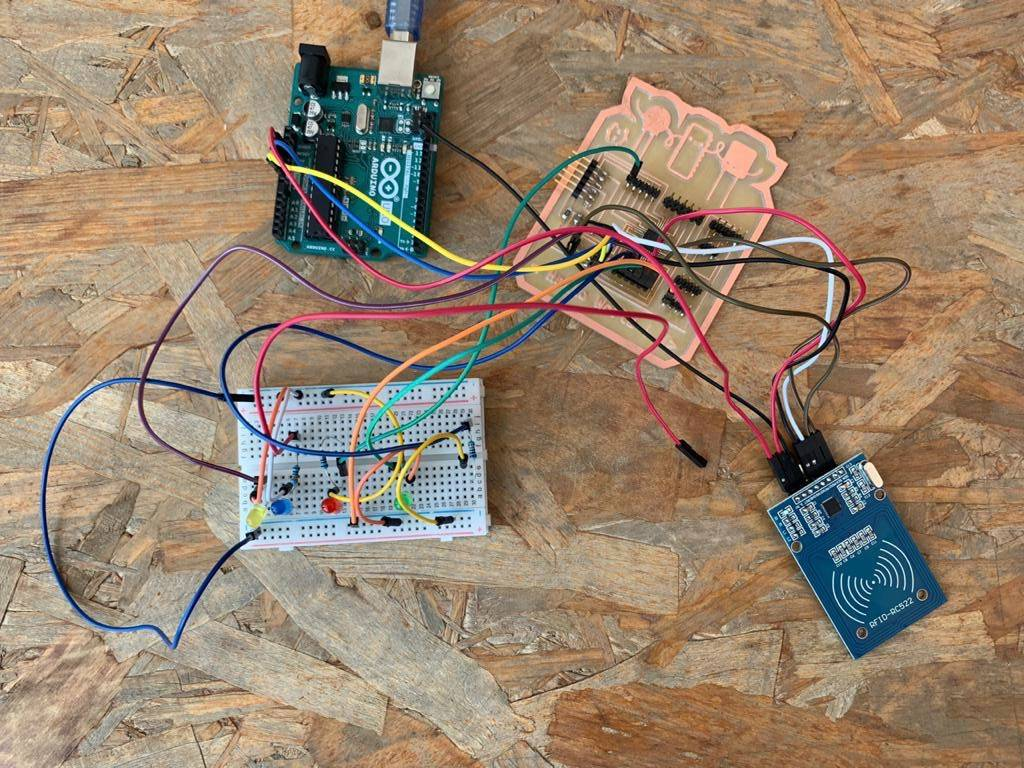#### PROGRAMMING

I followed some steps to write a code to light up an LED when the right tag will be detected.

Include the MRC522 Library

`#include `

Include the SPI Library to communicate via SPI with the Module.

`#include `

Define RFID Module SDA Pin that connected to the pin 10

`#define SS_PIN 10`

Define RFID Module RST Pin that connected to the pin 9

`#define RST_PIN 9`

Define LEDs that connected to the pins

`int LED_A = 8;`

`int LED_B = 7;`

`int LED_C = 6;`

`int LED_D = 5;`

`int LED_E = 4;`

Initialization of the library using the ARDUINO pins declared above.

`MFRC522 rfid(SS_PIN, RST_PIN);`

In the `the setup()`

Opens up the communication to the serial monitor in Arduino IDE

`Serial.begin(9600);`

`SPI.begin();`

Initialize MFRC522

`rfid.PCD_Init();`

Initialize digital pin LEDs as an output

`pinMode(LED_A, OUTPUT);`

`pinMode(LED_B, OUTPUT);`

`pinMode(LED_C, OUTPUT);`

`pinMode(LED_D, OUTPUT);`

`pinMode(LED_E, OUTPUT);`

In the `the loop()`

Look for new cards to select one.

`if (!rfid.PICC_IsNewCardPresent() || !rfid.PICC_ReadCardSerial())`

` return;`

`MFRC522::PICC_Type piccType = rfid.PICC_GetType(rfid.uid.sak);`

Check is the PICC of Classic MIFARE type

`if (piccType != MFRC522::PICC_TYPE_MIFARE_MINI &&`

`piccType != MFRC522::PICC_TYPE_MIFARE_1K &&`

`piccType != MFRC522::PICC_TYPE_MIFARE_4K) {`

`Serial.println(F("Your tag is not of type MIFARE`

`Classic."));`

`return;`

Store NUID into nuidPICC array

`String strID = "";`

`for (byte i = 0; i < 4; i++) {`

` strID +=`

`(rfid.uid.uidByte[i] < 0x10 ? "0" : "") +`

`String(rfid.uid.uidByte[i], HEX) +`

`(i!=3 ? ":" : "");`

`}`

`strID.toUpperCase();`

`Serial.print("Tap card key: ");`

`Serial.println(strID);`

check the card to light up the LED

`if (strID.indexOf("E2:F6:07:2E") >= 0) {`

`digitalWrite(LED_A, HIGH);`

`} else {`

`digitalWrite(LED_A, LOW);`

And make this step for all the LEDs and tags.

After the code was checked, I uploaded it to the PCB.

#### Any Problem ?

While working on different experiments using the Arduino, It became difficult for me to recognize the resistors values because it mixed up in the kit box.

#### What is a Resistor?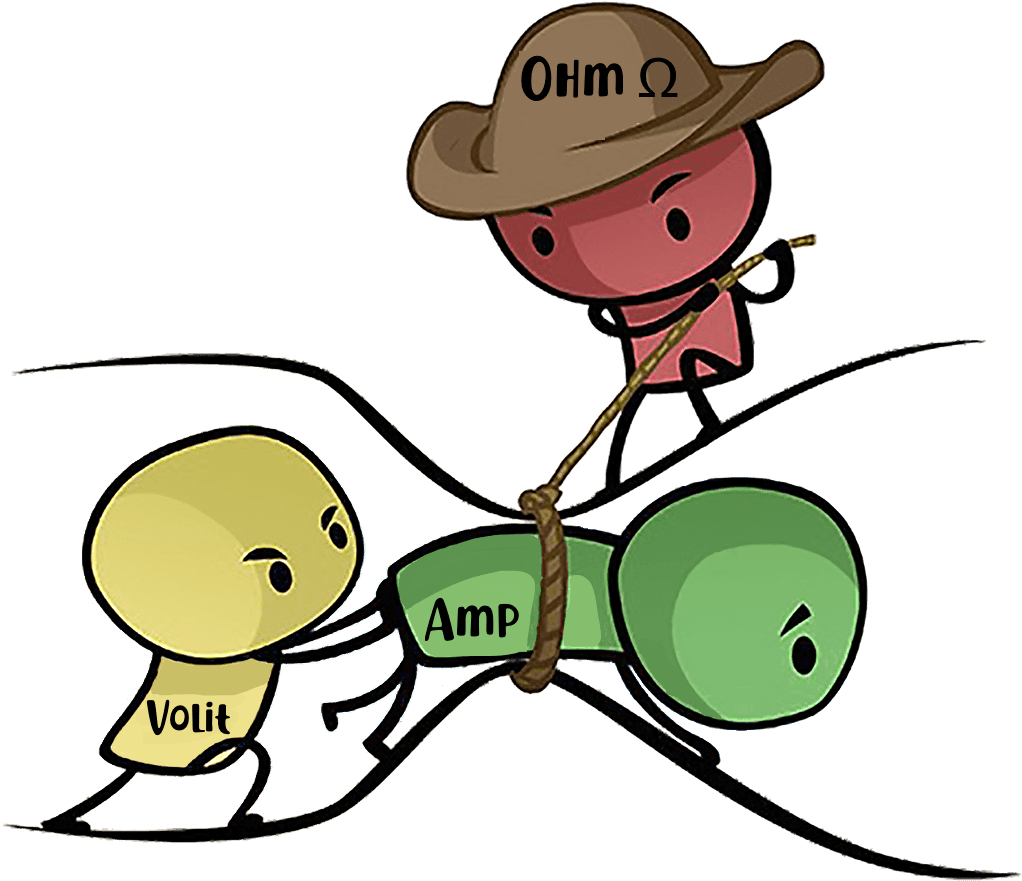Resistors are the passive components that used to reduce the flow of electric current in the electrical circuits.

#### What is a Tolerance ?

It is the percentage of error in the resistance value that says how much more or less one can expect a resistor's actual measured resistance to be from its stated resistance.

#### The Ohm’s Law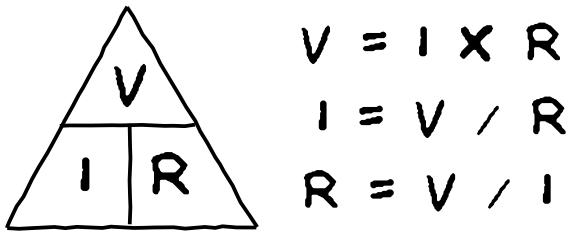This Law describes the relationship between the electrical current, voltage and resistance. Resistance is equal to Voltage divided over current.

#### HOW TO READ RESISTOR COLOR CODES ?

I used this chart to find the Resistor's value.

Also, this website helped me to calculate the value by selecting the number of bands then determining their colors.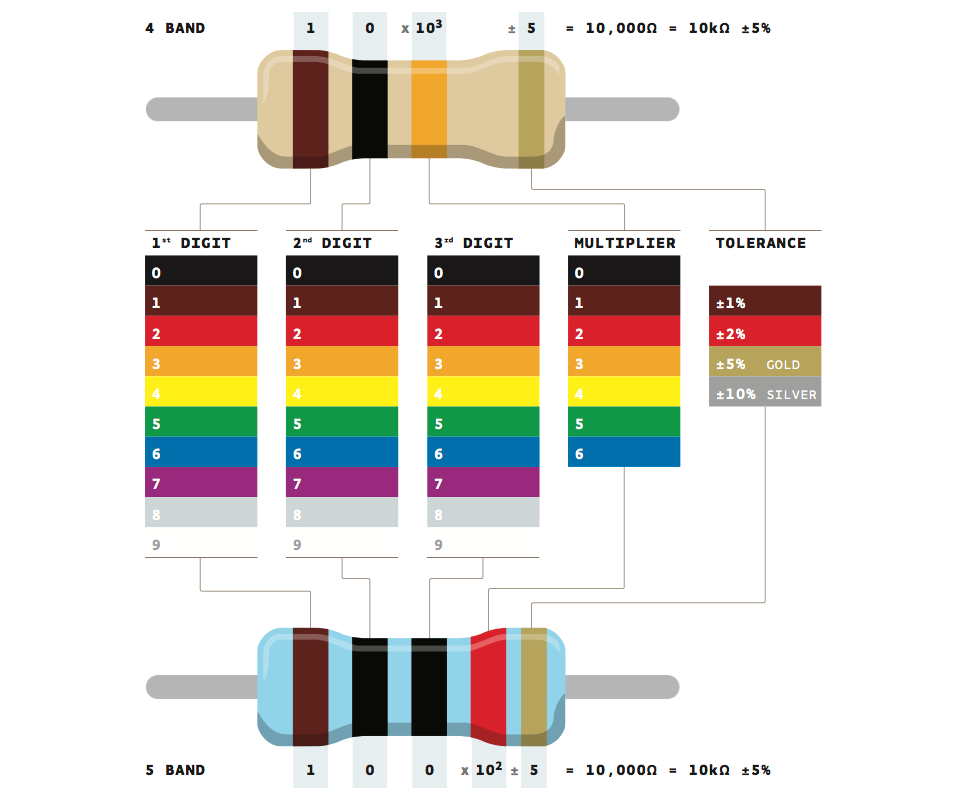Resistor has either 4 or 5 bands with different colors. Each color correlates to a specific number.

To read the Resistor value, we need to determine the gold(5%) or silver(10%) side which indicates the tolerance and start reading the resistor from the opposite side.

In the 4-band Resistor:

• The first two bands represent the first two digits of the Resistor.

• The third band represents the multiplier(the power of ten).

• The fourth band specifies the tolerance.

In the 5-band Resistor:

• The first three bands represent the first three digits of the Resistor.

• The fourth band represents the multiplier(the power of ten).

• The fifth band specifies the tolerance.

Code files:

LDR Code.

Ultrasonic Code.

RFID Code.

Resources:

Resistors

RFID with LED

Interface RC522 RFID Module